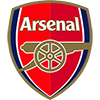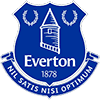2022 EPL Top Goalscorer of the Year Odds
-600
0.17 to 1Erling Haaland
48.1% implied probability

+1100
11 to 1Harry Kane
4.7% implied probability

+4400
44 to 1Gabriel Jesus
1.2% implied probability

+4400
44 to 1Mohamed Salah
1.2% implied probability

+4400
44 to 1Timo Werner
1.2% implied probability

No changes have been recorded yet.
+5000
50 to 1Aleksandar Mitrovic
1.1% implied probability

+5000
50 to 1Darwin Gabriel Nunez Ribeiro
1.1% implied probability

+6500
65 to 1Ivan Toney
0.9% implied probability

+8000
80 to 1Allan Saint-Maximin
0.7% implied probability

+8000
80 to 1Neal Maupay
0.7% implied probability

+8500
85 to 1Phil Foden
0.7% implied probability

+10000
100 to 1Dejan Kulusevski
0.6% implied probability

+10000
100 to 1Harvey Barnes
0.6% implied probability

+10000
100 to 1Leon Bailey
0.6% implied probability

+10000
100 to 1Bryan Mbeumo
0.6% implied probability

+10000
100 to 1Richarlison De Andrade
0.6% implied probability

+10000
100 to 1Eddie Nketiah
0.6% implied probability

+10000
100 to 1Jadon Sancho
0.6% implied probability

+10000
100 to 1Bruno Guimaraes Rodriguez Moura
0.6% implied probability

+10000
100 to 1Mason Mount
0.6% implied probability

+10000
100 to 1Pedro Neto
0.6% implied probability

+10000
100 to 1Gianluca Scamacca
0.6% implied probability

+10000
100 to 1Daniel Podence
0.6% implied probability

+10000
100 to 1Hee-Chan Hwang
0.6% implied probability

+10000
100 to 1Cristiano Ronaldo
0.6% implied probability

+10000
100 to 1Emile Smith Rowe
0.6% implied probability

+10000
100 to 1Julian Alvarez
0.6% implied probability

+10000
100 to 1Antony Matheus dos Santos
0.6% implied probability

+10000
100 to 1Alexander Isak
0.6% implied probability

+10000
100 to 1Taiwo Awoniyi
0.6% implied probability

+10000
100 to 1Armando Broja
0.6% implied probability

+10000
100 to 1Jesse Lingard
0.6% implied probability

+10000
100 to 1Deniz Undav
0.6% implied probability

+10000
100 to 1Ollie Watkins
0.6% implied probability

+10000
100 to 1Luis Diaz
0.6% implied probability

+10000
100 to 1Eberechi Eze
0.6% implied probability

+10000
100 to 1Joe Gelhardt
0.6% implied probability

+10000
100 to 1Brennan Johnson
0.6% implied probability

+10000
100 to 1Bukayo Saka
0.6% implied probability

+10000
100 to 1Patson Daka
0.6% implied probability

+10000
100 to 1Gabriel Martinelli
0.6% implied probability

+10000
100 to 1Dominic Calvert-Lewin
0.6% implied probability

+10000
100 to 1Che Adams
0.6% implied probability

+10000
100 to 1Odsonne Edouard
0.6% implied probability

+10000
100 to 1Jean-Philippe Mateta
0.6% implied probability

+10000
100 to 1Roberto Firmino
0.6% implied probability

+10000
100 to 1Jamie Vardy
0.6% implied probability

+10000
100 to 1Riyad Mahrez
0.6% implied probability

+10000
100 to 1Jack Grealish
0.6% implied probability

+10000
100 to 1Adam Armstrong
0.6% implied probability

+10000
100 to 1Heung-Min Son
0.6% implied probability

+10000
100 to 1Christian Eriksen
0.6% implied probability

+10000
100 to 1Wilfried Zaha
0.6% implied probability

+10000
100 to 1Danny Ings
0.6% implied probability

+10000
100 to 1Raheem Sterling
0.6% implied probability

+10000
100 to 1Christian Benteke
0.6% implied probability

No changes have been recorded yet.
+10000
100 to 1James Ward-Prowse
0.6% implied probability

+10000
100 to 1Michail Antonio
0.6% implied probability

+10000
100 to 1Kevin De Bruyne
0.6% implied probability

+10000
100 to 1Philippe Coutinho
0.6% implied probability

+10000
100 to 1Chris Wood
0.6% implied probability

+10000
100 to 1Raul Jimenez
0.6% implied probability

+10000
100 to 1Kai Havertz
0.6% implied probability

+10000
100 to 1James Maddison
0.6% implied probability

+10000
100 to 1Jarrod Bowen
0.6% implied probability

+10000
100 to 1Danny Welbeck
0.6% implied probability

+10000
100 to 1Nicolas Pepe
0.6% implied probability

+10000
100 to 1Diogo Jota
0.6% implied probability

+10000
100 to 1Bruno Miguel Borges Fernandes
0.6% implied probability

+10000
100 to 1Christian Pulisic
0.6% implied probability

+10000
100 to 1Jack Harrison
0.6% implied probability

+10000
100 to 1Bernardo Silva
0.6% implied probability

+10000
100 to 1Marcus Rashford
0.6% implied probability

+10000
100 to 1Rodrigo Moreno Machado
0.6% implied probability

+10000
100 to 1Kelechi Iheanacho
0.6% implied probability

+10000
100 to 1Patrick Bamford
0.6% implied probability

+10000
100 to 1Callum Wilson
0.6% implied probability

+10000
100 to 1Dominic Solanke
0.6% implied probability

+10000
100 to 1Anthony Martial
0.6% implied probability

+10000
100 to 1Pierre-Emerick Aubameyang
0.6% implied probability